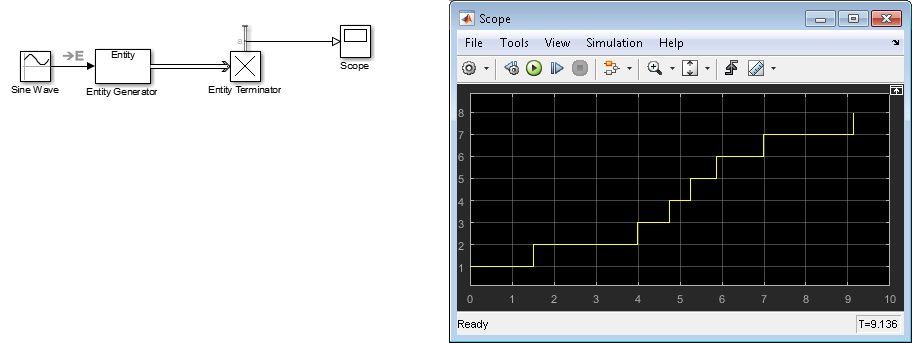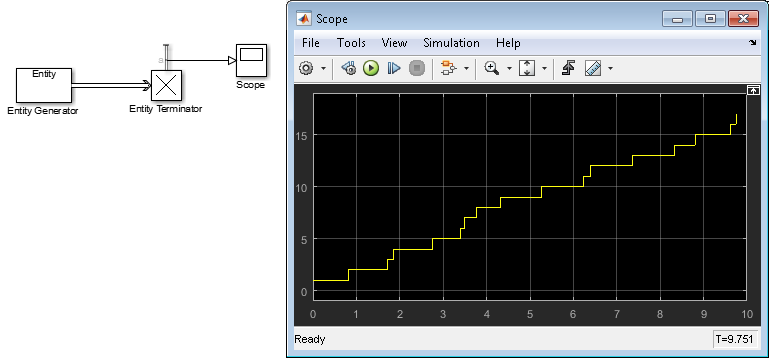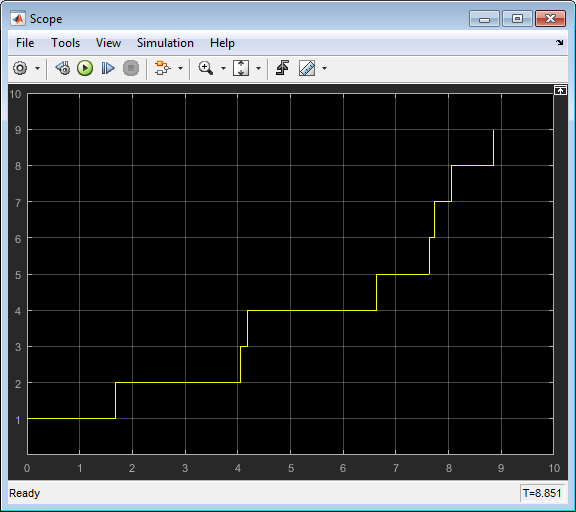## Specify Intergeneration Times for Entities

The intergeneration time is the time interval between successive entities that the block generates. You can have a generation process that is:

• Periodic

• Sampled from a random distribution or time-based signal

• From custom code

For example, if the block generates entities at T = 50, T = 53, T = 60, and T = 60.1, the corresponding intergeneration times are 3, 7, and 0.1. After each new entity departs, the block determines the intergeneration time that represents the interval until the block generates the next entity.

### Determine Intergeneration Time

You configure the Entity Generator block by indicating criteria that it uses to determine intergeneration times for the entities it creates. You can generate entities:

• From random distribution

• Periodically

• At arbitrary times

Use the dropdown list in the Time source parameter of the Entity Generation block to determine intergeneration times:

• `Dialog`

Uses the Period parameter to periodically vary the intergeneration times.

• `Signal port`

Uses a signal from an external block, such as the Sine wave block, to vary the intergeneration times.

• `MATLAB action`

Enables an Intergeneration time action field, in which you enter MATLAB® code to customize the intergeneration times.

#### Periodically Vary the Intergeneration Times

1. In a new model, from the SimEvents® library, drag the Entity Generator, Entity Terminator, and Scope blocks.

2. In the Entity Generation tab of the Entity Generator, set the Time source parameter to `Dialog`.

3. In the Statistics tab of the Entity Terminator block, select the Number of entities arrived check box.

4. Connect these blocks and simulate the model. The period is 1.5. Vary the period to `8` and simulate the model again. Observe the change in the scope.#### Use a Signal to Vary the Intergeneration Times

1. In a new model, from the SimEvents library, drag the Entity Generator and Entity Terminator blocks. From the Simulink® library add the Sine Wave, and Scope blocks.

2. In the Entity Generation tab of the Entity Generator, set the Time source parameter to `Signal port`.

A new signal port appears on the Entity Generator block.

3. In the Statistics tab of the Entity Terminator block, select the Number of entities arrived check box.

4. Double-click the Sine Wave block. By default, the first value of the Sine Wave block is `0`. To add a constant value to the sine to produce the output of this block, change the Bias parameter to another value, for example, `1.5`.

5. Connect these blocks and simulate the model.Upon generating each entity, the Entity Generator block reads the value of the input signal and uses that value as the time interval until the next entity generation.

Notice the capital E on the signal line from the Sine Wave block to the Entity Generator block. This icon indicates the transition from a time-based system to a discrete-event system.

#### Customize the Variation of the Intergeneration Times

1. In a new model, from the SimEvents library, drag the Entity Generator, Entity Terminator, and Scope blocks.

2. In the Entity Generation tab of the Entity Generator, set the Time source parameter to `MATLAB action`.

A new Intergeneration time action field appears on the Entity Generator block.

3. To customize the intergeneration times for your model, in the Intergeneration time action field, enter MATLAB code, for example:

`dt = rand();`

Note

For intergeneration times, you must set the fixed name, dt. You cannot set any other variable name for this value.

4. In the Statistics tab of the Entity Terminator block, select the Number of entities arrived check box.

5. Connect these blocks and simulate the model.To generate entities with exponential random arrival times, in the Intergeneration time action field, enter MATLAB code that uses the `mean` function, for example:

```mean = 1; dt = -mean*log(1-rand());```## Related Examples